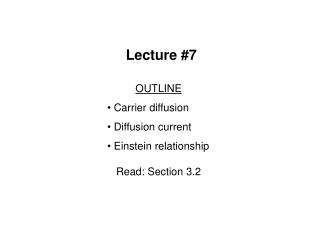DownloadDownload PresentationLecture #7

# Lecture #7

Télécharger la présentation## Lecture #7

- - - - - - - - - - - - - - - - - - - - - - - - - - - E N D - - - - - - - - - - - - - - - - - - - - - - - - - - -
##### Presentation Transcript

1. Lecture #7 OUTLINE Carrier diffusion Diffusion current Einstein relationship Read: Section 3.2

2. Diffusion Particles diffuse from regions of higher concentration to regions of lower concentration region, due to random thermal motion. EE130 Lecture 7, Slide 2

3. 1-D Diffusion Example • Thermal motion causes particles to move into an adjacent compartment every t seconds • Each particle has an equal probability of jumping to the left and to the right. EE130 Lecture 7, Slide 3

4. Diffusion Current x x D is the diffusion constant, or diffusivity. EE130 Lecture 7, Slide 4

5. Total Current J= JN + JP JN = JN,drift + JN,diff = qnne+ JP = JP,drift + JP,diff = qppe– EE130 Lecture 7, Slide 5

6. The position of EF relative to the band edges is determined by the carrier concentrations, which is determined by the dopant concentrations. In equilibrium, EF is constant; therefore, the band energies vary with position: Non-Uniformly-Doped Semiconductor Ec(x) EF Ev(x) EE130 Lecture 7, Slide 6

7. In equilibrium, there is no net flow of electrons or holes  The drift and diffusion current components must balance each other exactly. (A built-in electric field exists, such that the drift current exactly cancels out the diffusion current due to the concentration gradient.) JN = 0 and JP = 0 e dn = + = m J qn qD 0 N n N dx EE130 Lecture 7, Slide 7

8. Consider a piece of a non-uniformly doped semiconductor: Ec(x) EF n e = - q kT Ev(x) EE130 Lecture 7, Slide 8

9. Einstein Relationship between D and m Under equilibrium conditions,JN = 0 andJP = 0 e dn = + = m J qn qD 0 N n N dx qD e e = - m N 0 qn qn n kT Similarly, Note: The Einstein relationship is valid for a non-degenerate semiconductor, even under non-equilibrium conditions EE130 Lecture 7, Slide 9

10. Example: Diffusion Constant What is the hole diffusion constant in a sample of silicon with p = 410 cm2 / V s ? Solution: Remember: kT/q = 26 mVat room temperature. EE130 Lecture 7, Slide 10

11. The ratio of carrier densities (n, p) at two points depends exponentially on the potential difference between these points: Potential Difference due to n(x), p(x) EE130 Lecture 7, Slide 11

12. Quasi-Neutrality Approximation • If the dopant concentration profile varies gradually with position, then the majority-carrier concentration distribution does not differ much from the dopant concentration distribution. • n-type material: • p-type material: • in n-type material EE130 Lecture 7, Slide 12

13. Summary • Electron/hole concentration gradient  diffusion • Current flowing in a semiconductor is comprised of drift & diffusion components for electrons & holes • In equilibrium, JN = JN,drift + JN,diff = 0 • The characteristic constants of drift and diffusion are related: J= JN,drift + JN,diff + JP,drift + JP,diff EE130 Lecture 7, Slide 13